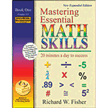#### Mean - Median - Mode - RangeDo you have a challenge remembering what to do to find the mean, median, mode, and range? Here is a refresher and tips to remember which math process to use.

I. Mean
II. Median
III Mode
IV. Range

I. Mean
If you know how to find the average of a set of numbers, then you know how to find the mean. They are the same. The average or mean is found by adding the numbers to find the sum. Then, divide the sum by how many numbers were added.

Example: Find the mean of 20, 4, 6, 7, and 13

Step 1. Add the numbers: (20 + 4 + 6 + 7 + 13 = 50)
Step 2. Divide the sum by how many numbers were added. The sum is 50. Five numbers were added. 50 divided by 5 equals ten. (50 / 5 = 10)
Therefore, the mean of 20, 4, 6, 7, and 13 is 10

II. Median

What do you think of when you here the word, median, outside of a math class? Middle? Okay, notice the first three letters, “med.” “MED” usually stands for medium, and is the size between small and large. So, think of the middle number when you think of median. I hope that works for you.

Now, let us find the middle number in the above set of numbers. Again the numbers were 20, 4, 6, 7, and 13.

Step 1. – Put the numbers in ascending order.
4, 6, 7, 13, 20

Step 2. - Identify the middle number.
The middle number is 7.

Therefore, the median is 7.

It is easy to identify the middle number if the set contains an odd number.
What if the set has an even amount of numbers?

Find the median for 20, 4, 6, 7, 13, and 11.

Step 1. Put the numbers in ascending order.
4, 6, 7, 11, 13, 20

Step 2. Identify the two middle numbers. The two middle numbers are 7 and 11.

Step 3. Find the average of the two numbers in step 2.
11 + 7 = 18
18 / 2 = 9

The median for 20, 4, 6, 7, 13, and 11, is 9.

III. Mode
Sometimes on Wednesdays, my day mostly consist of cleaning house. So, I could say that I was in a domestic mode. If I see you wear orange 4 out of 5 days in a week, I could say you are in an orange mode. In other words, mode is the number repeated the most times. The best way to identify the mode in a set of numbers is to do the following.

Find the Mode of 20, 4, 6, 20, 13, 20, and 11

Step 1. – Put numbers in ascending order
4, 6, 11, 13, 20, 20, 20

Step 2. - The number that occurs most frequently is the mode.

The mode for 20, 4, 6, 20, 13, 20, 11, is 20.

IV. Range

Ordinarily, the word, range, represents the distance from one point to another point. Similarly, the range for a set of numbers is found by subtracting the smallest number from the largest number.

Find the range for the numbers: 20, 4, 6, 20, 13, 20, and 11

Step 1. – Put numbers in ascending order
4, 6, 11, 13, 20, 20, 20

Step 2. – subtract the smallest number from the largest number
20 – 4 = 16

The range for 20, 4, 6, 20, 13, 20, and 11, is 16.

So, practice! You may purchase a book with short concise practice lessons below from
Christianbook.com HomeMastering Essential Math Skills, Revised Edition: Book One Produce motivated and confident math students with this innovative, easy-to-use system of teaching basic math! Each daily lesson contains five key components: two speed drills, review exercises, Helpful Hints, new material and a daily word problem. Lessons are not rigid however; teachers have the flexibility to modify lesson plans while structure and a basic framework are still provided. Eight chapters cover whole numbers, fractions, decimals, percentages, integers, geometry, charts, graphs and problem solving. Help your students really master the essential math skills they'll need throughout their lives with enthusiasm and consistency. Answer key and final review included. 142 pages, softcover. For Grades 4-5. Book 1.

Exponent Basics
Converting Improper Fractions and Mixed Numbers
Prime FactorizationRelated Articles
Editor's Picks Articles
Top Ten Articles
Previous Features
Site Map